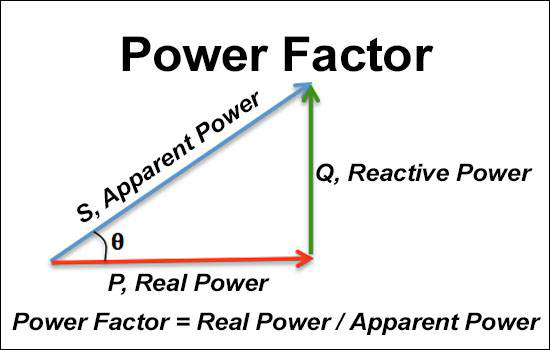# Power Factor Calculator

#### By R.W. Hurst, EditorA power factor calculator is a tool used to calculate the power factor pf of an electrical circuit. PF measures how effectively electrical power is being used in a circuit. It is the ratio of the real power (in watts) to the circuit's apparent power (in volt-amperes).

A power factor calculator typically takes as input the values of the real power and the apparent power in an ac circuit and then calculates the PF by dividing the real power by the apparent power. The resulting PF is a decimal value between 0 and 1, with higher values indicating a more efficient use of electrical power.

A high PF is important because it indicates that the circuit uses electrical power efficiently, which can result in lower energy costs and reduced stress on the electrical infrastructure. Therefore, it can be useful for engineers, electricians, and anyone working with electrical circuits to ensure their systems operate efficiently.

What does a power factor calculator calculate?

It is a tool that calculates the PF of an electrical circuit. PF measures how efficiently electrical power is being used in a circuit. It is the ratio of the real power (in watts) to the circuit's apparent power (in volt-amperes).

The calculator takes as input the values of the real power and the apparent power in a circuit, which can be measured using a power meter or a multimeter, and then calculates the PF by dividing the real power by the apparent power.

The real power in a circuit represents the power consumed by the electrical load, such as a motor or a heating element. The apparent power is the product of the circuit's voltage and current and represents the total power supplied to the load. The difference between the real power and the apparent PF of a circuit is called reactive power, which is the active power not directly used by the load but stored and released by reactive components.

Calculating the PF, line-to-line voltage, volt-amperes, real work, and power factors required can be determined. Apparent power reactive power can also be calculated. It can provide insight into how efficiently a circuit uses electrical power and help identify opportunities to improve energy efficiency and reduce electricity costs. A high PF indicates that the circuit is using power efficiently. In contrast, a low PF indicates that the circuit is using power inefficiently and may need optimization.

Steps to calculate power factor

Here are the steps to calculate power factor.

1. Determine the real power (in watts) consumed by the load. This can be measured using a power meter or a multimeter.

2. Determine the apparent power (in volt-amperes) supplied to the load. This can be calculated by multiplying the voltage (in volts) by the circuit's current (in amperes).

3. Divide the real power by the apparent power to obtain the PF. The PF formula is Power factor = Real power / Apparent power.

4. The PFwill be a decimal value between 0 and 1. A PF of 1 means that the circuit is using electrical power efficiently, while a PF less than 1 indicates that the circuit is using power inefficiently due to the presence of reactive components in the circuit.

It's worth noting that PF correction can be performed to improve the PF of a circuit. This can be achieved by adding PF correction capacitors or by using equipment with a higher PF. A higher PF can reduce energy costs, increase system capacity, and improve voltage regulation.

Power factor correction

PF correction is the process of improving the PF of an electrical circuit by adding reactive components, typically capacitors, to the circuit. Power factor correction can be calculated using the following steps:

1. Determine the existing PF of the circuit.

2. Determine the desired PF for the circuit. A PF of 1 is ideal, but a specific target PF may be desired depending on the load and the electrical infrastructure.

3. Calculate the reactive power of the circuit. This difference between the apparent power and the real power in the circuit represents the power being stored and released by reactive components. The formula for reactive power is: Reactive power = Apparent power x sin(θ), where θ is the angle between the voltage and the current in the circuit.

4. Calculate the amount of capacitance needed for PF correction. This can be calculated using the following formula: Capacitance = (Reactive power x PF correction factor) / (2π x voltage^2), where the PF correction factor is the difference between the desired PF and the existing PF.

5. Install the appropriate capacitors to correct the PF of the circuit. The capacitance value and the installation method will depend on the specific circuit and the electrical infrastructure.

It's important to note that true PF correction should only be performed after a thorough electrical system analysis and only by qualified electricians or engineers. Incorrect PF correction can lead to over-correction and other power quality issues, resulting in equipment damage or safety hazards.

Poor PF refers to a situation where the PF of an electrical single-phase circuit is less than 1, indicating that the circuit needs to be using electrical power efficiently. This can be due to the presence of reactive components in the circuit, such as inductive loads like motors or transformers, which cause the current to lag behind the voltage in the circuit.

• 1
• 2
• 3
• 4
• 5
• 6
• 7
• 8
• 9
• 10
• 11
• 12
• 13
• 14
• 15
• 16
• 17
• 18
• 19
• 20
• 21
• 22
• 23
• 24
• 25
• 26
• 27
• 28
• 29
• 30
• 31

Top